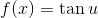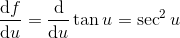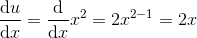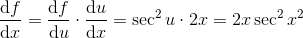# AP Calculus AB : Understanding the derivative of trigonometric functions

## Example Questions

### Example Question #1 : Understanding The Derivative Of Trigonometric Functions

Find the derivative of the following function: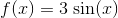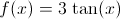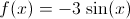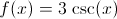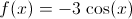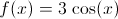Explanation:

The derivative of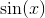is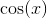. It is probably best to memorize this fact (the proof follows from the difference quotient definition of a derivative).

Our functionthe factor of 3 does not change when we differentiate, therefore the answer is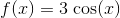### Example Question #2 : Understanding The Derivative Of Trigonometric Functions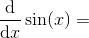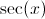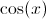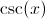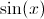Explanation:

The derivative of a sine function does not follow the power rule. It is one that should be memorized.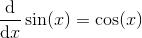.

### Example Question #3 : Understanding The Derivative Of Trigonometric Functions

What is the second derivative of?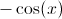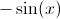Explanation:

The derivatives of trig functions must be memorized. The first derivative is:.

To find the second derivative, we take the derivative of our result.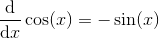.

Therefore, the second derivative will be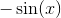.

### Example Question #21 : Calculus Ii — Integrals

Find the derivative of the function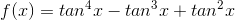.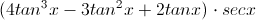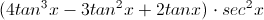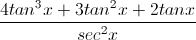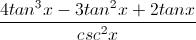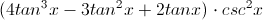Explanation:

We can use the Chain Rule:

Let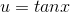, so that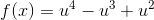.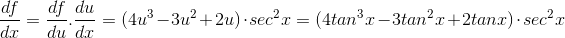### Example Question #5 : Understanding The Derivative Of Trigonometric Functions

Compute the derivative of the function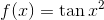.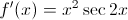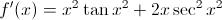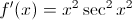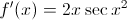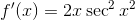Explanation:

Use the Chain Rule.

Set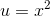and substitute.